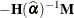Morel (1989) and Morel, Bokossa, and Neerchal (2003) suggested a bias correction of the classical sandwich estimator that rests on an additive correction of the residual crossproducts and a sample size correction. This estimator is available with the EMPIRICAL=MBN option in the PROC GLIMMIX statement. In the notation of the previous section, the residual-based MBN estimator can be written as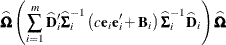where

•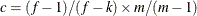or c = 1 when you specify the EMPIRICAL=MBN(NODF) option

• f is the sum of the frequencies

• k equals the rank of•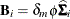•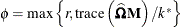•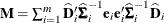•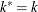if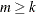, otherwise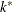equals the number of nonzero singular values of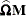•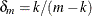if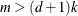and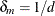otherwise

•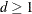and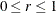are parameters supplied with the mbn-options of the EMPIRICAL=MBN(mbn-options) option. The default values are d = 2 and r = 1. When the NODF option is in effect, the factor c is set to 1.

Rearranging terms, the MBN estimator can also be written as an additive adjustment to a sample-size corrected classical sandwich estimatorBecause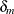is of order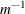, the additive adjustment to the classical estimator vanishes as the number of independent sampling units (subjects) increases. The parameteris a measure of the design effect (Morel, Bokossa, and Neerchal, 2003). Besides good statistical properties in terms of Type I error rates in small-m situations, the MBN estimator also has the desirable property of recovering rank when the number of sampling units is small. If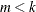, the meat piece of the classical sandwich estimator is essentially a sum of rank one matrices. A small number of subjects relative to the rank ofcan result in a loss of rank and subsequent loss of numerator degrees of freedom in tests. The additive MBN adjustment counters the rank exhaustion. You can examine the rank of an adjusted covariance matrix with the COVB(DETAILS) option in the MODEL statement.

When the principle of the MBN estimator is applied to the likelihood-based empirical estimator, you obtain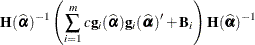where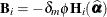, and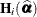is the second derivative of the log likelihood for the ith sampling unit (subject) evaluated at the vector of parameter estimates,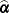. Also,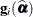is the first derivative of the log likelihood for the ith sampling unit. This estimator is computed if you request EMPIRICAL=MBN with METHOD=LAPLACE or METHOD=QUAD.

In terms of adjusting the classical likelihood-based estimator (White 1982), the likelihood MBN estimator can be written asThe parameteris determined as

•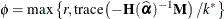•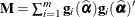•if, otherwiseequals the number of nonzero singular values of